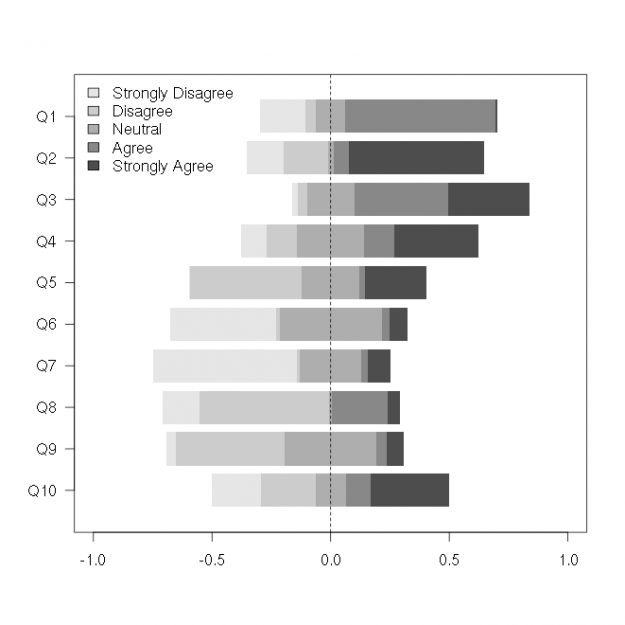# Recipe for Centered Horizontal Stacked Barplots (Useful for Likert scale responses)There is a nice package and paper about this here: http://www.jstatsoft.org/v57/i05/paper. However, the associated code is complex and uses lattice. Here's a brief recipe using base graphics that implements the above figure:

``````
set.seed(40)
x <- matrix(rgamma(50,1,1),10,5)
x <- x/rowSums(x)
colnames(x) <- c("Strongly Disagree", "Disagree",
"Neutral", "Agree", "Strongly Agree")
rownames(x) <- paste0("Q", 1:nrow(x))

## colors for each category
clrs <- rev(gray.colors(ncol(x))) ## colors

## centering category
acat <- 3 ## "Neutral"

## separation between bars
sepr <- 0.2

## ncol and nrow
nr <- nrow(x)
nc <- ncol(x)

## reorder so that questions 1:nrow(x) go from top down
x <- x[nr:1,]

## compute center offsets
cnof <- apply(x, 1, function(y) {
lo <- if(acat > 1) sum(y[1:(acat-1)]) else 0
hi <- sum(y[1:acat])
lo + (hi-lo)/2
})

## create plot
plot(c(-1,1), c(1,nr), type="n",
ylim=c(1-(1-sepr)/2-sepr,
nr+(1-sepr)/2+sepr),
ylab="", yaxt="n",
xlab="", )

## plot bars
for(i in 1:nr) {
for(j in 1:nc) {
lo <- if(j > 1) sum(x[i,][1:(j-1)]) else 0
hi <- sum(x[i,][1:j])
polygon(x=c(lo, lo, hi, hi)-cnof[i],
y=c(i-(1-sepr)/2, i+(1-sepr)/2,
i+(1-sepr)/2, i-(1-sepr)/2),
col=clrs[j], border=NA)
}
}

## create y-axis
axis(2, at=1:nr, las=2, xpd=NA, labels=rownames(x))
legend("topleft", fill=clrs, bty="n", legend=colnames(x))

abline(v=0, lty=2)
``````

## 5 thoughts on “Recipe for Centered Horizontal Stacked Barplots (Useful for Likert scale responses)”

1.gd047 says:

Another solution can be found here: http://jason.bryer.org/likert/

2.Jason Bryer says:

Check out the likert package for a ggplot2 implementation of this. More info here: http://jason.bryer.org/likert

3.BioStatMatt says: